• 机器人运动学方程的D-H表示法，应用最为广的确定机器人空间位置的算法。
• 为研究带柔索双臂巡检机器人越障过程中手臂与箱体的运动规律,采用D-H参数法建立了机器人基本越障动作的连杆坐标系,推导出了机器人运动学方程;以机器人蠕动越障过程为例,结合相关约束条件分析计算了越障过程不同阶段...
• 利用运动弹性动力理论及有限元方法，分析了柔性并联机器人各支链的弹性变形、弹性位移及其耦合关系，提出了柔性并联机器人运动约束条件和动力约束条件，建立了平面柔性并联机器人的系统方程。以平面3-RRR柔性...
• 机器人运动估计系列（二）——运动学方程 前言 在上一篇文章中，我们了解了用于表示机器人位置、速度的坐标系的定义，学习如何表示姿态，也就是旋转的三种表达方式：旋转矩阵、欧拉角以及四元数。在这一节的第一...
机器人运动估计系列（二）——运动学方程（上）

前言

在上一篇文章中，我们了解了用于表示机器人位置、速度的坐标系的定义，学习了如何表示姿态，也就是旋转的三种表达方式：旋转矩阵、欧拉角以及四元数。在这一节的第一部分中，我们将继续讨论在三种旋转表达之间互相转换的方法。之后，我们将以四麦轮小车以及四旋翼为例，推导二者的运动学模型，并分享一些我在学习过程中的一些心得体会。

1 各旋转表达方式的转换

1.1 欧拉角 - - > 旋转矩阵

在上一篇文章中，我们定义了在这个系列里欧拉角的旋转顺序与方式，即Z-Y-X，并且是内在旋转。下面，我们来推导由欧拉角到旋转矩阵的转换关系。

1、绕Z轴旋转 ψ$\psi$ 角度（从n系到1系）
C1n=⎡⎣⎢cosψ−sinψ0sinψcosψ0001⎤⎦⎥C_{n}^{1} =  \left[
\begin{matrix}
\cos{\psi} & \sin{\psi} & 0 \\
-\sin{\psi} & \cos{\psi} & 0 \\
0 & 0 & 1 \\
\end{matrix}
\right]

2、绕Y轴旋转 θ$\theta$ 角度（从1系到2系）
C21=⎡⎣⎢cosθ0sinθ010−sinθ0cosθ⎤⎦⎥C_{1}^{2} =  \left[
\begin{matrix}
\cos{\theta} & 0 & -\sin{\theta} \\
0 & 1 & 0 \\
\sin{\theta} & 0 & \cos{\theta} \\
\end{matrix}
\right]

3、绕X轴旋转 γ$\gamma$ 角度（从2系到b系）
Cb2=⎡⎣⎢1000cosγ−sinγ0sinγcosγ⎤⎦⎥C_{2}^{b} =  \left[
\begin{matrix}
1 & 0 & 0 \\
0 & \cos{\gamma} & \sin{\gamma} \\
0 & -\sin{\gamma} & \cos{\gamma} \\
\end{matrix}
\right]

4、结合三次旋转推导出完整的旋转矩阵
Cbn=Cb2⋅C21⋅C1nC_{n}^{b} = C_{2}^{b} \cdot C_{1}^{2} \cdot C_{n}^{1}
Cbn=⎡⎣⎢cosθcosψsinθsinγcosψ−cosγsinψsinθcosγcosψ+sinγsinψcosθsinψsinθsinγsinψ+cosγcosψsinθcosγsinψ−sinγcosψ−sinθcosθsinγcosθcosγ⎤⎦⎥C_{n}^{b} = \left [
\begin{matrix}
\cos{\theta} \cos{\psi} & \cos{\theta} \sin{\psi} & -\sin{\theta} \\
\sin{\theta} \sin{\gamma} \cos{\psi} - \cos{\gamma} \sin{\psi} & \sin{\theta} \sin{\gamma} \sin{\psi} + \cos{\gamma} \cos{\psi} & \cos{\theta} \sin{\gamma} \\
\sin{\theta} \cos{\gamma} \cos{\psi} + \sin{\gamma} \sin{\psi} & \sin{\theta} \cos{\gamma} \sin{\psi} - \sin{\gamma} \cos{\psi} & \cos{\theta} \cos{\gamma}
\end{matrix}
\right ]

以上便定义了由欧拉角到旋转矩阵的转换关系。但是注意一点，这个旋转矩阵的含义是指：将一个在n系下表示的向量，投影到b系下进行表示。这里，n系指的是世界系或者说是导航系，b系指的是体坐标系。

1.2 四元数 - - > 旋转矩阵

根据上一篇文章的描述，要利用四元数表示由一个三维向量 un$\boldsymbol{u^{n}}$ 得到向量  ub$\boldsymbol{u^{b}}$ ，方法如下：
ub=q∗un∗q−1 \boldsymbol{u^{b}}=q* \boldsymbol{u^{n}}*q^{-1}
其中，q=[q0 q1 q2 q3]$q=[q_{0} \ q_{1} \ q_{2} \ q_{3}]$。
根据四元数乘法法则，有：
ub=M(q)⋅M′(q−1)⋅un \boldsymbol{u^{b}}=M(q) \cdot M^{'}(q^{-1})\cdot \boldsymbol{u^{n}}
其中，M(q)$M(q)$代表的是四元数的左乘矩阵，M′(q−1)$M^{'}(q^{-1})$代表的是四元数的右乘矩阵。两个矩阵的定义在这里就不细说了，最终可以得到的结果就是：
M(q)⋅M′(q−1)=⎡⎣⎢⎢⎢⎢××××0q21+q20−q23−q222(q1q2+q0q3)2(q1q3−q0q2)02(q1q2−q0q3)q22−q23+q20−q212(q2q3+q0q1)02(q1q3+q0q2)2(q2q3−q0q1)q23−q22−q21+q20⎤⎦⎥⎥⎥⎥M(q) \cdot M^{'}(q^{-1})=\left [
\begin{matrix}
\times & 0 & 0 & 0 \\
\times & q_{1}^{2}+q_{0}^{2}-q_{3}^{2}-q_{2}^{2} & 2(q_{1}q_{2}-q_{0}q_{3}) & 2(q_{1}q_{3}+q_{0}q_{2}) \\
\times & 2(q_{1}q_{2}+q_{0}q_{3}) & q_{2}^{2}-q_{3}^{2}+q_{0}^{2}-q_{1}^{2} & 2(q_{2}q_{3}-q_{0}q_{1}) \\
\times &  2(q_{1}q_{3}-q_{0}q_{2}) & 2(q_{2}q_{3}+q_{0}q_{1}) & q_{3}^{2}-q_{2}^{2}-q_{1}^{2}+q_{0}^{2}\\
\end{matrix}
\right ]
所以，
ub=Cbn⋅un \boldsymbol{u^{b}}=\boldsymbol{C}_{n}^{b}\cdot \boldsymbol{u^{n}}
Cbn=⎡⎣⎢⎢q21+q20−q23−q222(q1q2+q0q3)2(q1q3−q0q2)2(q1q2−q0q3)q22−q23+q20−q212(q2q3+q0q1)2(q1q3+q0q2)2(q2q3−q0q1)q23−q22−q21+q20⎤⎦⎥⎥\boldsymbol{C}_{n}^{b}=\left [
\begin{matrix}
q_{1}^{2}+q_{0}^{2}-q_{3}^{2}-q_{2}^{2} & 2(q_{1}q_{2}-q_{0}q_{3}) & 2(q_{1}q_{3}+q_{0}q_{2}) \\
2(q_{1}q_{2}+q_{0}q_{3}) & q_{2}^{2}-q_{3}^{2}+q_{0}^{2}-q_{1}^{2} & 2(q_{2}q_{3}-q_{0}q_{1}) \\
2(q_{1}q_{3}-q_{0}q_{2}) & 2(q_{2}q_{3}+q_{0}q_{1}) & q_{3}^{2}-q_{2}^{2}-q_{1}^{2}+q_{0}^{2}\\
\end{matrix}
\right ]

1.3 四元数 - - > 欧拉角

根据上面的推导，可以发现旋转矩阵作为中间桥梁衔接了几种不同的旋转表达方式。通过欧拉角能够推出对应的旋转矩阵，通过四元数也能够推出对应的旋转矩阵，所以欧拉角和四元数的对应关系也就变得清晰明了了。通过四元数可以得到欧拉角，通过欧拉角也能求出四元数。这里以四元数得到欧拉角举例：
根据上面的推导，很容易得到：
⎧⎩⎨⎪⎪⎪⎪⎪⎪⎪⎪⎪⎪−sinθ=2(q1q3+q0q2)cosθcosψ=q21+q20−q23−q22cosθsinψ=2(q1q2−q0q3)cosθsinγ=2(q2q3−q0q1)cosθcosγ=q23−q22−q21+q20\begin{cases}
-\sin{\theta} =  2(q_{1}q_{3}+q_{0}q_{2})\\
\cos{\theta} \cos{\psi}=q_{1}^{2}+q_{0}^{2}-q_{3}^{2}-q_{2}^{2}  \\
\cos{\theta} \sin{\psi}=2(q_{1}q_{2}-q_{0}q_{3}) \\
\cos{\theta} \sin{\gamma} = 2(q_{2}q_{3}-q_{0}q_{1}) \\
\cos{\theta} \cos{\gamma}  = q_{3}^{2}-q_{2}^{2}-q_{1}^{2}+q_{0}^{2}
\end{cases}
所以，可以得到：
θ=arcsin[−2(q1q3+q0q2)]\theta = \arcsin \left[ -2(q_{1}q_{3}+q_{0}q_{2}) \right]
ψ=arctan[2(q1q2−q0q3)q21+q20−q23−q22]\psi = \arctan \left[\frac{2(q_{1}q_{2}-q_{0}q_{3})}{q_{1}^{2}+q_{0}^{2}-q_{3}^{2}-q_{2}^{2} } \right]
γ=arctan[2(q2q3−q0q1))q23−q22−q21+q20]\gamma = \arctan \left[\frac{2(q_{2}q_{3}-q_{0}q_{1}))}{ q_{3}^{2}-q_{2}^{2}-q_{1}^{2}+q_{0}^{2} } \right]
需要注意的是，在机器人的运动中，俯仰角θ$\theta$都是定义在−π/2$-\pi/2$到π/2$\pi/2$之间的，所以cosθ$\cos{\theta}$大于0。由此也可以推出，其余两个角在[0 2π]$[0 \ 2\pi]$范围内的具体大小。

2 运动学方程的引入

运动学主要是研究物体的位置、速度、角速度、加速度、角加速度等特征之间的关系。有了运动学方程，就能很容易的从其中的某些量出发推导得到另一些量，比如可以测量物体加速度和角速度就能根据这二者积分解算出位置和速度（惯性导航的基本原理）。本文将以四麦轮机器人以及四旋翼无人机为例，简单的介绍一下运动学方程是如何建立的。

要学习构造运动学方程，首先要理解一点，同一个运动在不同坐标系下的描述是不相同的。

举个例子，假如一个物体P相对于坐标系A下以速度[10,10,0]$[10,10,0]$运动，而坐标系B是A系绕Z轴经过180度的旋转得到的，那么这个物体的速度在B系中的表示就应该是[−10,−10,0]$[-10,-10,0]$。理解了这一点后，我们可以思考一个稍微复杂一些的问题，当B系相对与A系存在一个平移速度[1,1,0]$[1,1,0]$时，这个物体的速度在B系下的表示就应该是
[−10,−10,0]−[−1,−1,0]=[−9,−9,0][-10,-10,0]-[-1,-1,0]=[-9,-9,0]
这也很好理解，那么要是B系不仅与A系之间有平移速度还有旋转角速度呢，这个应该怎么表示？这时就轮到哥氏定理闪亮登场了！

2.1 理解哥氏定理

这部分内容主要参考一个介绍刚体转动动力学的PPT。
哥氏定理描述的是一般的空间自由质点相对于不同坐标系的速度和加速度的相互转换。尤其是当两个坐标系之间存在相对旋转时，需要格外注意。
下文中推导了哥氏定理的具体形式。
假设有一个坐标系n与一个坐标系b，另外定义一个向量r$\boldsymbol{r}$，如下：
r=rx⋅ib+ry⋅jb+rz⋅kb\boldsymbol{r}=r_{x} \cdot \boldsymbol{i}_{b}+r_{y} \cdot \boldsymbol{j}_{b}+r_{z} \cdot \boldsymbol{k}_{b}
将该向量在n系下求导，即：
dnrdt=dndt(rx⋅ib+ry⋅jb+rz⋅kb)\frac{d_{n}\boldsymbol{r}}{dt}=\frac{d_{n}}{dt}
\left (
r_{x} \cdot \boldsymbol{i}_{b}+r_{y} \cdot \boldsymbol{j}_{b}+r_{z} \cdot \boldsymbol{k}_{b} \right )

dnrdt=dnrxdt⋅ib+dnrydt⋅jb+dnrzdt⋅kb+rx⋅dnibdt+ry⋅dnjbdt+rz⋅dnkbdt\frac{d_{n}\boldsymbol{r}}{dt}=
\frac{d_{n} r_{x}}{dt} \cdot \boldsymbol{i}_{b} +
\frac{d_{n} r_{y}}{dt} \cdot \boldsymbol{j}_{b} +
\frac{d_{n} r_{z}}{dt} \cdot \boldsymbol{k}_{b} +
r_{x} \cdot \frac{d_{n} \boldsymbol{i}_{b}}{dt} +
r_{y} \cdot \frac{d_{n} \boldsymbol{j}_{b}}{dt} +
r_{z} \cdot \frac{d_{n} \boldsymbol{k}_{b}}{dt}

又因为，当两个参考系之间存在相对转动时，有
dnibdt=ωnb×ib\frac{d_{n} \boldsymbol{i}_{b}}{dt}=\boldsymbol{\omega}_{nb} \times \boldsymbol{i}_{b}
dnjbdt=ωnb×jb\frac{d_{n} \boldsymbol{j}_{b}}{dt}=\boldsymbol{\omega}_{nb} \times \boldsymbol{j}_{b}
dnkbdt=ωnb×kb\frac{d_{n} \boldsymbol{k}_{b}}{dt}=\boldsymbol{\omega}_{nb} \times \boldsymbol{k}_{b}
所以，最终可以得到下面等式：
dnrdt=dbrdt+ωnb×r\frac{d_{n}\boldsymbol{r}}{dt}=\frac{d_{b}\boldsymbol{r}}{dt}
+ \boldsymbol{\omega}_{nb}  \times \boldsymbol{r}

根据上面的推导可知，同一个向量相对两个不同的坐标系对于时间求导，只有在两个坐标系没有相对旋转时才会相等，否则应该运用哥氏定理来求解。

另外，关于哥氏定理，有两点需要注意！！！
第一点，在上面推导中用到的向量r$\boldsymbol{r}$既可以代表速度向量也可以代表位置向量。
例如，将r$\boldsymbol{r}$用速度向量v$\boldsymbol{v}$替代，则有：
dnvdt=dbvdt+ωnb×v\frac{d_{n}\boldsymbol{v}}{dt}=\frac{d_{b}\boldsymbol{v}}{dt} + \boldsymbol{\omega}_{nb}  \times \boldsymbol{v}
第二点，必须理解：
dnrdt≠Cnb⋅dbrdt\frac{d_{n}\boldsymbol{r}}{dt} \ne  \boldsymbol{C}_{b}^{n} \cdot \frac{d_{b}\boldsymbol{r}}{dt}
事实上，左边这一项称为绝对导数，是指向量r$\boldsymbol{r}$在n系下的时间变化率在b系下的投影，右边这一项是相对导数，指的是向量r$\boldsymbol{r}$在b系下的时间变化率在b系下的投影。两者实际上都是表示在b系下的，不存在上面这个等式的关系。另外，正是由于n系和b系之间存在相对转动，才会导致向量r$\boldsymbol{r}$在两个系中的时间变化率不相同，而与两个坐标系之间的相对角速度有关。

3 四麦轮小车运动学模型的构建

在前文中，我们花了很大的篇幅介绍了坐标系的定义、旋转的表达、甚至引入了哥氏定理，这一切都是在为我们建立小车的运动学模型打下基础。下面就从麦克纳姆轮的性质开始，首先探讨了轮速与小车车速的关系，之后讨论了小车车速与加速度、位置之间的关系。

3.1 轮速运动学模型

本部分内容主要参考了一篇知乎专栏《麦克纳姆轮浅谈》。
在这篇博文中，作者介绍了麦克纳姆轮的前世今生，也通过一系列的推导，得出了四麦克纳姆轮小车的轮速与小车车速之间的关系，在这里就不多啰嗦啦，直接使用相关结论，大家感兴趣可以移步去看那一篇博文。

四麦轮小车的结构如图：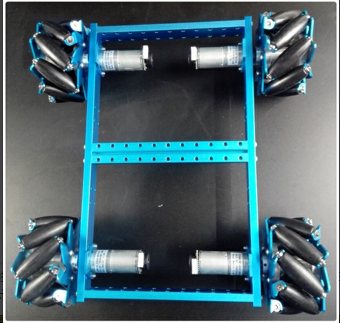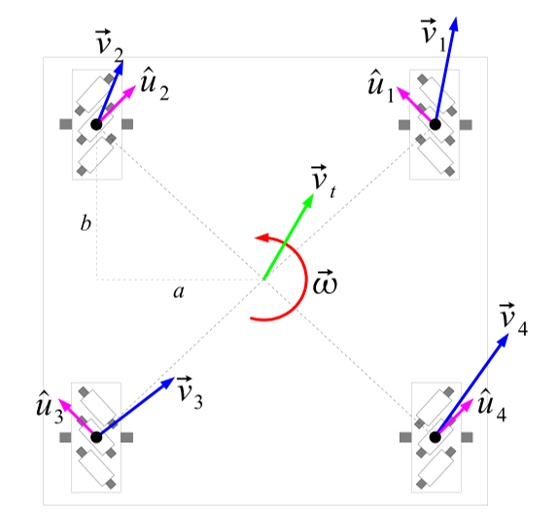小车轮速与车速的关系如下：
⎡⎣⎢⎢⎢ω1ω2ω3ω4⎤⎦⎥⎥⎥=⎡⎣⎢⎢⎢⎢1111−11−11a+b−(a+b)−(a+b)a+b⎤⎦⎥⎥⎥⎥⎡⎣⎢vxvywz⎤⎦⎥\left[ \begin{matrix} \omega_{1} \\
\omega_{2} \\
\omega_{3} \\
\omega_{4}  \end{matrix} \right ] =
\left [ \begin{matrix}
1 & -1 & a+b \\
1 & 1 & -(a+b) \\
1 & -1 & -(a+b) \\
1 & 1 & a+b
\end{matrix} \right ]
\left [ \begin{matrix}
v_{x} \\
v_{y} \\
w_{z}
\end{matrix} \right ]

其中，ω1$\omega_{1}$等代表轮1到轮4的旋转角速度，而vx,vy$v_{x},v_{y}$代表小车在体坐标系下的X、Y轴速度，ωz$\omega_{z}$代表小车Z轴旋转角速度。上式是小车的逆运动学模型，而小车的正运动学模型，可以通过上面的方程中抽取三个解出来，或者使用最小二乘法的到，在这里不再赘述。

3.2 速度运动学模型

在上一部分中，我们介绍了轮速与车速之间的关系。这个运动学方程主要作用是通过码盘测量轮速，然后间接得到小车车速。而在这一部分的运动学模型推导中，主要就是为了建立小车的加速度、角速度与速度和位移之间的关系。（因为加速度和角速度能够通过IMU测量得到）

参考下面这幅图，定义了导航系n以及小车的体坐标系b（图中未表示的Z轴的方向：垂直纸面向里）。由于大多数情况下，小车还是在平地上运动的，这里假设小车做二维运动。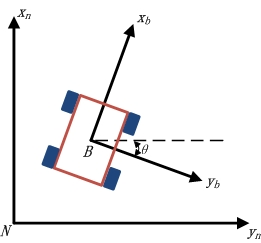所以，首先可以推出b系与n系之间的旋转关系。由于是在二维平面上的旋转，不必考虑复杂的欧拉角，可以直接得到旋转矩阵，如下：
Cbn=[cosθ−sinθsinθcosθ]\boldsymbol{C}_{n}^{b}=
\left [ \begin{matrix}
\cos{\theta} & \sin{\theta} \\
-\sin{\theta} & \cos{\theta}
\end{matrix} \right ]

根据牛顿定律，位置是速度的一阶积分，速度是加速度的一阶积分，所以有：
p˙n=Cbn⋅vb\dot{\boldsymbol{p}}^{n}=\boldsymbol{C}_{n}^{b} \cdot \boldsymbol{v}^{b}
v˙b=dnvbdt−ωnb×vb\dot{\boldsymbol{v}}^{b}=\frac{d_{n}\boldsymbol{v}^{b}}{dt} - \boldsymbol{\omega}_{nb}  \times \boldsymbol{v}^{b}
θ˙=ωz\dot{\theta}=\omega_{z}
其中，pn$\boldsymbol{p}^{n}$代表小车在导航系n中的位置，vb$\boldsymbol{v}^{b}$代表小车相对于n系的速度在b系下的表示，ωnb$\boldsymbol{\omega}_{nb}$代表b系相对与n系的旋转角速度，θ$\theta$代表b系与n系的夹角（见上图），ωz$\omega_{z}$代表ωnb$\boldsymbol{\omega}_{nb}$在Z轴上的分量。

上面三个式子中，第一个很简单，先把b系下表示的速度通过旋转矩阵投影到n系下，然后积分得到位置。第三个式子也很简单，就是角速度的积分等于角度。而在第二个式子中则用到前文提到的哥氏定理，但是这里产生了一个疑问？

问题：
为什么这里不写成：
v˙b=dbvbdt\dot{\boldsymbol{v}}^{b}=\frac{d_{b}\boldsymbol{v}^{b}}{dt}
而是要写成：
v˙b=dnvbdt−ωnb×vb\dot{\boldsymbol{v}}^{b}=\frac{d_{n}\boldsymbol{v}^{b}}{dt} - \boldsymbol{\omega}_{nb}  \times \boldsymbol{v}^{b}

这个形式呢？

回答：
这和传感器能够测量到的量有关。在机器人领域最常用的加速度计传感器只能测量到的是比力，也就是单位质量上作用的非引力外力。用更通俗的话来说，加速度计不能区分载体的运动加速度和引力加速度，只能测量载体相对于惯性空间的绝对加速度和引力加速度之和。用数学的语言表示就是：
f=dnvbdt−G\boldsymbol{f}=\frac{d_{n}\boldsymbol{v}^{b}}{dt}-\boldsymbol{G}
其中，f$\boldsymbol{f}$代表加速度计测量到的比力，G$\boldsymbol{G}$代表重力矢量，中间的微分项代表相对于惯性空间的绝对加速度（因为我们在第一节中就已经假设了n系是惯性系）。

简单的说就是，我们通常只能测到：
dnvbdt\frac{d_{n}\boldsymbol{v}^{b}}{dt}
而很难测到：
dbvbdt\frac{d_{b}\boldsymbol{v}^{b}}{dt}
所以我们把第二个运动学方程式写成这样。我们还能进一步把运动学方程写为：
v˙b=f−ωnb×vb+G\dot{\boldsymbol{v}}^{b}=\boldsymbol{f} - \boldsymbol{\omega}_{nb}  \times \boldsymbol{v}^{b} +\boldsymbol{G}

3.3 运动学模型汇总

在上面两部分的描述后，我们建立了轮速与车速之间的运动学模型，并且建立了车速与加速度、速度、角度、角速度之间的运动学模型。基于这两个运动学模型，我们就能够方便的通过诸如码盘、加速度计、陀螺仪等传感器测量得到轮速、加速度、角速度等，然后再推算出位置、速度、姿态等等余下的状态量。

两个模型的最终形式如下。
1、轮速-车速模型：

⎡⎣⎢vbxvbywz⎤⎦⎥=(JT⋅J)−1JT⎡⎣⎢⎢⎢ω1ω2ω3ω4⎤⎦⎥⎥⎥
\left [ \begin{matrix}
v_{x}^{b} \\
v_{y}^{b} \\
w_{z}
\end{matrix} \right ] =
(\boldsymbol{J}^{T} \cdot \boldsymbol{J})^{-1}\boldsymbol{J}^{T}
\left[ \begin{matrix} \omega_{1} \\
\omega_{2} \\
\omega_{3} \\
\omega_{4}  \end{matrix} \right ]

其中，
J=⎡⎣⎢⎢⎢⎢1111−11−11a+b−(a+b)−(a+b)a+b⎤⎦⎥⎥⎥⎥
\boldsymbol{J}=
\left [ \begin{matrix}
1 & -1 & a+b \\
1 & 1 & -(a+b) \\
1 & -1 & -(a+b) \\
1 & 1 & a+b
\end{matrix} \right ]

2、加速度-速度-位置模型：
⎧⎩⎨⎪⎪⎪⎪⎪⎪⎪⎪⎪⎪p˙n=Cbn⋅vbv˙b=f−ωnb×vb+Gθ˙=ωz\begin{cases}
\dot{\boldsymbol{p}}^{n}=\boldsymbol{C}_{n}^{b} \cdot \boldsymbol{v}^{b} \\
\\
\dot{\boldsymbol{v}}^{b}=\boldsymbol{f} - \boldsymbol{\omega}_{nb}  \times \boldsymbol{v}^{b} +\boldsymbol{G} \\
\\
\dot{\theta}=\omega_{z}
\end{cases}

将式中的向量都分别展开，得到：
⎧⎩⎨⎪⎪⎪⎪⎪⎪⎪⎪⎪⎪⎪⎪⎪⎪⎪⎪⎪⎪⎪⎪⎪⎪⎪⎪⎪⎪⎪⎪⎪⎪p˙nx=cosθ⋅vbx+sinθ⋅vbyp˙ny=−sinθ⋅vbx+cosθ⋅vbyv˙bx=fbx+ωz⋅vbyv˙by=fby−ωz⋅vbxθ˙=ωz\begin{cases}
\dot{{p}}_{x}^{n}=\cos{\theta} \cdot v_{x}^{b} +  \sin{\theta} \cdot v_{y}^{b} \\
\\
\dot{{p}}_{y}^{n}=-\sin{\theta} \cdot v_{x}^{b} +  \cos{\theta} \cdot  v_{y}^{b} \\
\\
\dot{{v}}_{x}^{b}=f_{x}^{b}+\omega_{z} \cdot v_{y}^{b}\\
\\
\dot{{v}}_{y}^{b}=f_{y}^{b}-\omega_{z} \cdot v_{x}^{b}\\
\\
\dot{\theta}=\omega_{z}
\end{cases}

其中，由于假设了小车在水平面上运动，所以重力不影响X和Y轴上的加速度。另外，各个变量的含义前文中也介绍得很清楚了，不再复述。

小结

这篇文章中，首先介绍了旋转之间的互相转换。这里貌似还看不出它们的作用，但是在下一篇介绍四旋翼运动学模型的文章中，你将会看到它们有多么重要。之后，引入了很重要的哥氏定理。这主要是因为加速度计的测量值包括两部分：相对惯性空间的绝对加速度以及引力加速度。而相对惯性空间的绝对加速度中包含了由于物体转动引起的附加加速度，只有去除这部分有害加速度，才能积分得到物体相对与惯性系的速度在物体系下的投影（需要仔细理解一下哥氏定理）。最后，讨论了四麦轮小车的运动学模型。

限于篇幅，四旋翼无人机运动学模型的构建将放在下一篇文章中介绍。由于从二维空间进入了三维空间，关于旋转的部分理解起来难度会加大，另外也将会引入重要的姿态更新方法，敬请期待啦！！！
展开全文• 机器人运动学教程，详细讲解了机器人坐标的建立、DH方程的求解，以及运动学的正逆解
• 根据机器人D-H矩阵理论,通过VS50机器人工件中心坐标系向机座坐标系的齐次坐标变换,建立了该机器人运动学正问题的数学模型。求解该方程并测量该模型在运动仿真前后其工件中心的位置,使VS50机器人的数学模型和运动仿真...
• 这是台湾科大教授的机器人运动学课程1，有在研究机器人方面需要用到的机器人结构以及机器人运动方程解析的同学，可以用到，此番资料，大陆网络找不到哟！
• 耐噪ZD设计公式在时变非线性方程求解中在机器人运动学控制中的应用研究论文
• 第一步，求解一般形式的一系列连接臂的运动学和动力学方程。 这是通过 Matlab 的符号数学工具箱完成的。 第二步，在Matlab的虚拟现实环境中模拟上一步的结果。matlab
• 这是台湾科大教授的机器人运动学课程3，有在研究机器人方面需要用到的机器人结构以及机器人运动方程解析的同学，可以用到，此番资料，大陆网络找不到哟！
• 运用D-H参数法建立了测温机器人运动学方程,对机器人末端执行器的运动进行理论推导,并利用ADAMS软件对机器人进行运动学仿真研究,最后利用测温机器人物理样机对运动过程进行测试。结果表明,机器人结构设计合理,运动...
• 首先建立了月面坐标系中月球机器人的直角坐标运动学方程, 并在此基础上推导出运动学方 程的极坐标表达形式。然后,针对月球机器人的运动控制问题,推导了为保证月球机器人的运动全局渐 近收敛其线速度和...
• 运动学方程为基础,设计了柔性臂空间机器人的惯性空间内连续轨迹规划算法。仿真表明,规划的机械臂关节运动规律可以补偿柔性连杆振动给机械臂末端位置带来的影响,使机械臂末端位置准确沿着期望的 轨迹运动。
• 针对履带驱动方式具有非完整约束的性质,利用李群理论和微分几何的分析方法,对各种构型情况下灵巧壁面移动机器人运动学和动力学特性进行了研究,证明了灵巧壁面移动机器人的可控性,建立了机器人动力学方程,为机器人...
• 有关机器人运动学方面的教程，详细介绍了制作机器人时，有关运动方程的建立和解答。
• 三、机器人运动学 从机械的角度看，一个机械手可以用一系列通过转动关节或移动关节连接的刚体（连杆）运动链进行概要表示。链的一端安装在基座上，末端执行器则安装在链的另一端。链结构的运动结果可以通过每一...
三、机器人运动学

从机械的角度看，一个机械手可以用一系列通过转动关节或移动关节连接的刚体（连杆）运动链进行概要表示。链的一端安装在基座上，末端执行器则安装在链的另一端。链结构的运动结果可以通过每一连杆相对前一连杆的基本运动合成得到。因而，为了在空间中操作目标，有必要描述出末端执行器的位置和方向。本节基于线性代数知识，通过系统而一般性的方法，推导得出正运动学方程（direct kinematics equation），使末端执行器的位置和方向（简称为位姿）可以表示为相对参考坐标系机械结构中关节变量的函数。其中开链和闭链运动学结构都将考虑。为获得方向的最简表示（minimal representation of orientation），本节将介绍操作空间（operational space）的概念，并建立其与关节空间（joint space）的关系。

3.1  刚体的姿态

刚体（rigid body）可以由其在空间中相对参考坐标系的位置和方向（简记为位姿）进行完整的描述。如图所示，令为标准正交参考坐标系，为坐标轴的单位向量。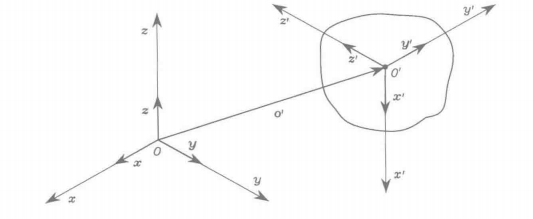图3.1  刚体的位置和方向

刚体上的点相对坐标系的位置可以表示为关系式

其中，表示向量在坐标轴上的分量。的位置可以简写为（）向量

（3.1）

由于除了方向和模长，其作用点和作用线都是规定的，因此是有界向量。

为了描述刚体的指向，考虑建立一个固连于刚体的标准正交坐标系，并由其相对参考坐标系的单位向量来表示。令此坐标系为中的表达式为：

（3.2）

每一单位向量的分量都是坐标系的轴相对参考坐标系的方向余弦。

3.2  旋转矩阵

为了描述简便起见，上式中描述刚体相对参考坐标系的指向的三个单位向量可以组合为一个（）矩阵。

（3.3）

定义R为旋转矩阵。

需要注意矩阵R的列向量相互正交，原因在于它们表示的是正交坐标系的单位向量，即

同时，其模长均为1，即

因此，矩阵R是一个正交矩阵，即

（3.4）

其中，表示（）单位矩阵。

如果在上式的两边同时右乘逆矩阵，可以得到以下有用的结论：

（3.5）

即旋转矩阵的转置与其逆矩阵相等。进一步地，注意到如果坐标系满足右手法则，则det()=1，如果满足左手法则，则det()=-1。

如上定义的旋转矩阵属于实（）矩阵中的特殊正交群（special orthonormal group），其列为正交的且行列式为1。在作空间旋转时，m=3，在作平面旋转时，m=2。

3.2.1  基本旋转

考虑一个坐标系可以通过参考坐标系相对某一坐标轴的基本旋转得到。如果想对坐标轴作逆时针方向旋转，则旋转为正。

假设参考坐标系绕轴旋转角度，令为旋转后的坐标系。新坐标系的单位向量可以通过其相对参考坐标系的分量来描述。即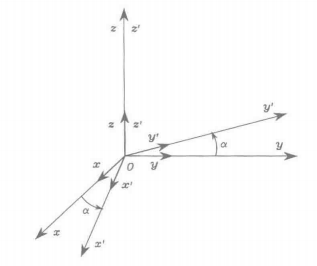图3.2  坐标系绕坐标轴旋转角

从而，坐标系 关于坐标系的旋转矩阵为

（3.6）

类似的，绕轴旋转角及绕轴旋转的旋转可以分别由下列式子给出

（3.7）

（3.8）

这些矩阵将有助于描述绕空间中任一轴的旋转。

容易验证，上述几式中的基本旋转具有如下性质：

（3.9）

考虑上式，旋转矩阵可以被赋予几何意义，即矩阵R描述了在空间中将参考坐标系的坐标轴调整到与机器人本体坐标系相应的坐标轴相一致时，所需要绕单个坐标轴进行的旋转。

3.2.2  向量的表示

为更深入理解旋转矩阵的几何含义，考虑本体坐标系原点与参考坐标系原点重合的情况（图3.3），此时，，其中代表零向量。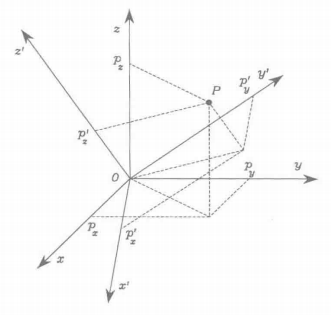图3.3  点在两个不同坐标系中的表示

相对于坐标系，空间中的一点可以表示为

或相对于坐标系，点可以表示为

由于和表示的是同一点，有

而且，根据式（3.3），有

（3.10）

旋转矩阵表示坐标系中的向量坐标转换为同一向量在坐标系中的坐标的变换矩阵（transformation matrix）。由式（3.4）的正交性质，逆变换可简单地由下式给出

（3.11）

3.2.3  向量的旋转

旋转矩阵也可视为使某一向量绕空间中任一轴旋转给定角度的矩阵算子。事实上，令为参考坐标系中的一个向量，由于矩阵的正交性，乘积得到的向量与的模相等，但是按照矩阵关于进行了旋转。模相等这一点可以通过并利用式（3.4）加以证明。后面还将对旋转矩阵的这一解释进行讨论。

3.3  旋转矩阵的合成

为了推导旋转矩阵的合成规则，有必要考虑一个向量在两个不同参考坐标系下的表示。因此，令、、为三个具有同一原点的坐标系。描述空间中任一点位置的向量可以在上述三个坐标系中进行表示。令分别代表向量在三个坐标系中的表示。

首先，考虑向量在坐标系2中的表达式与同一个向量在坐标系1中的表达式之间的关系。如果代表坐标系关于坐标系的旋转矩阵，有

（3.12）

类似地，有

（3.13）

（3.14）

另一方面，将式（3.12）代入式（3.13），并利用式（3.14）得到

（3.15）

式（3.15）中的矩阵关系可以解释为连续旋转的合成。考虑一个坐标系初始状态与坐标系一致。用矩阵表示的旋转可以看作是通过两步得到的：

首先根据对给定坐标系进行旋转，使之与坐标系一致。
然后根据将现在已与坐标系一致的坐标系进行旋转，使之与坐标系一致。
注意到完整的旋转可以表示成一系列的部分旋转，每一个部分旋转均相对前一个旋转进行定义，进行旋转的参考坐标系定义为当前坐标系。从而按给定的旋转顺序右乘旋转矩阵，进行连续旋转的合成，如式（3.15）。采用式（3.5）中的记法，有

（3.16）

连续旋转也可以一直相对初始坐标系来进行解释。在此情形下，旋转是相对固定坐标系进行的。令为坐标系关于固定坐标系的旋转矩阵，然后令表征坐标系相对坐标系0的矩阵关系，它由坐标系1根据矩阵进行旋转得到。由于（3.15）式给出了绕当前坐标系的轴进行连续旋转的合成规则，整体旋转可以被看作是通过以下步骤得到的：

首先用坐标系0通过旋转对坐标系1进行重排。
然后，将当前坐标系的旋转表示为。
最后，通过逆旋转补偿重排坐标系进行的旋转。
由于上述旋转是关于当前坐标系进行描述的，应用式（3.15）的合成规则可得

考虑式（3.16），有

（3.17）

其中，得到的不同于式（3.15）中的矩阵。由此可知，关于固定坐标系连续旋转的合成，可以通过按照给定的旋转顺序，左乘单个旋转矩阵进行。

回顾旋转矩阵在当前坐标系相对固定坐标系的指向方面的含义，可以意识到，旋转矩阵的列为当前坐标系的轴相对固定坐标系的方向余弦，而其行（其转置矩阵和逆矩阵的列）则为固定坐标系的轴关于当前坐标系的方向余弦。

旋转合成中的一个重要问题，是矩阵乘法不满足交换律。考虑到这一点，可以得出这样的结论，即一般来说两个旋转不可交换顺序，并且其合成关系依赖于单个旋转的顺序。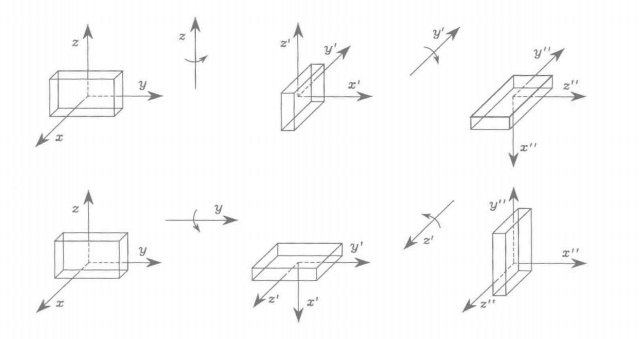图3.6  物体绕当前坐标系的轴的相继旋转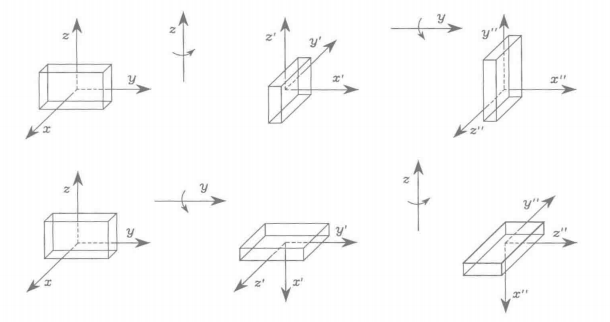图3.7  物体绕固定坐标系的轴的连续旋转

3.4  欧拉角

旋转矩阵对于坐标系指向的描述是冗余的。旋转矩阵用了9个元素来刻画其特征，而事实上，由于式（3.4）给出的正交性条件带来6个约束，这9个元素之间不是独立的，而是相关的。这就意味着，只要3个参数就足以描述一个刚体在空间中的指向。由3个独立的参数对指向进行描述，构成最简表示式。事实上，特殊正交群的最简表示需要个参数，因此，需要用3个参数来表示，而表示一个平面旋转只需要一个参数。

指向的最简表示可以通过三个角度的集合得到。将表示绕一个坐标轴进行基本旋转的旋转矩阵看成是单个角度的函数。这样，一般的旋转矩阵就可以通过3个基本旋转的1个适当序列的合成来实现，在此过程中需要保证两个连续旋转不是绕平行轴进行的。这就意味着在27种可能的组合中，只有12种不同的角度集合是可行的，每一集合表示3个一组的欧拉角。下面，将对两组欧拉角进行分析，即和（或滚动-俯仰-偏航）角。

3.4.1  角

用角描述的旋转可以通过如下的基本旋转合成得到。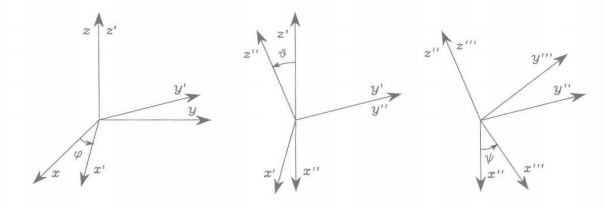图3.8  欧拉角的表示

将参考坐标系绕轴旋转角度，这一旋转可以用式（3.6）定义的矩阵来描述。
将当前坐标系绕轴旋转角度，这一旋转可以用式（3.7）定义的矩阵来描述。
将当前坐标系绕轴旋转角度，这一旋转同样可以用式（3.6）定义的矩阵来描述。
最终坐标系的指向通过相对当前坐标系旋转的合成得到，因此可以通过右乘基本旋转矩阵来计算，即

（3.18）

确定相应于给定旋转矩阵的欧拉角集合，对逆运动学问题求解很有用。假定旋转矩阵为

将此表达式与式（3.18）中的表达式相比较。考虑元素和，假定及，有

其中，为两个自变量的反正切函数。然后，求元素和的平方和并利用元素，得到

选择项的系数为正，将的取值范围限定在。基于这样的假设， 考虑元素和，有

故，所求解为

（3.19）

也有可能得到其他的解，这些解与（3.19）式中的解效果是一样的。在中选择得到

（3.20）

当=0时，解（3.19）、（3.20）会退化。在这种情形下，可能只能确定和的和或差。事实上，如果，，则连续的旋转和是绕当前坐标系的平行轴进行的，这就使得旋转是等价的。

3.4.2  角

另一组欧拉角来源于（航空）航海领域方向的表示。这些是角，也称为滚动-俯仰-偏航角，用来指示飞行器姿态的典型改变。在这种情形下，角表示相对固连于飞行器质心的固定工坐标系定义的旋转。（图3.4）

按照滚动-俯仰-偏航角得到的旋转结果可以按照如下步骤获得：

将参考坐标系绕轴旋转角度（偏航角），这一旋转由如（3.8）式定义的矩阵描述。
将参考坐标系绕轴旋转角度（俯仰角），这一旋转由如（3.7）式定义的矩阵描述。
将参考坐标系绕轴旋转角度（滚动角），这一旋转由如（3.6）式定义的矩阵描述。
坐标系的最终指向由相对固定坐标系旋转的合成得到，因此可以通过左乘基本旋转矩阵计算得到，即

（3.21）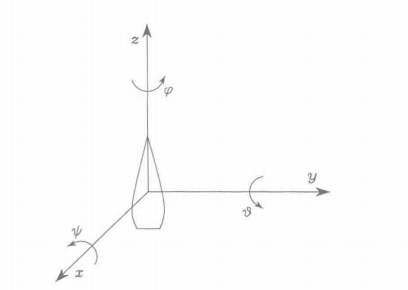图3.9  滚动-俯仰-偏航角度的表示

而欧拉角是如下给定旋转矩阵的逆解

可以通过将其与式（3.21）中的表达式相比较得到。

当属于区间时，有

（3.22）

当属于区间时时，其等价的解为

（3.23）

当时，得到（3.22）和（3.23）中的退化解。此时，有可能只能确定和的和或差。

3.5  角和轴

绕空间中某一轴旋转指定角度的指向的非最简表达式，可以采用4个参数进行表示。这一点在机械手末端执行器指向的轨迹规划问题中是有用的。

令为关于参考坐标系的旋转轴的单位向量。为了导出表示绕轴旋转角度的旋转矩阵，方便的做法是对绕参考坐标系的坐标轴的基本旋转进行合成。如果旋转是绕轴逆时针方向进行的，则角度为正。

如图3.10所示，一个可能的解决方案是首先将轴旋转必要的角度，使之与轴一致，然后绕轴旋转度，最后旋转必要的角度使单位向量与初始方向一致。具体的，旋转序列如下所示，其中旋转始终是相对固定坐标系的轴进行的：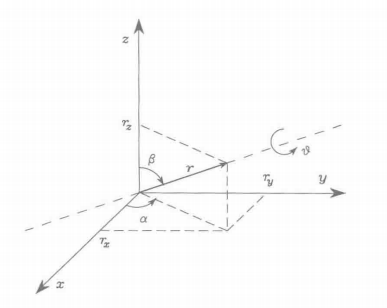图3.10  绕一轴旋转一定的角度

使得与一致，方法是先绕转角，再绕转角。
绕转角。
重排使与的初始指向一致，方法是先绕转角，再绕转角。
归纳起来，最终的旋转矩阵为

（3.24）

通过单位向量的分量，可以提取出需要用于计算式（3.24）中旋转矩阵的超越函数，以便消除对和的依赖。实际上，有

于是可以发现相应于给定角度和轴的旋转矩阵为

（3.25）

该矩阵具有如下的性质：

（3.26）

即，绕旋转与绕旋转没有区别，因此，这种表示不是唯一的。

如果需要求问题的逆解以计算相应于如下给定旋转矩阵的轴和角度

以下结论是有用的：

（3.27）

（3.28）
其中，注意表达式（3.27）、（3.28）是通过4个变量来描述旋转的，即角度量和轴的单位向量的3个分量。但是，易知的3个分量不是独立的，而是受到以下条件的约束

（3.29）

如果，表达式（3.27）和（3.28）将失去意义。为了求解逆问题，有必要直接参考通过旋转矩阵得到的特定表达式，并找到和情形下的求解公式。注意到，当（不旋转）时，单位向量是任意的。

3.6  单位四元数

角度/轴表达式的不足可以通过一个不同的四参数表达式加以克服。即单位四元数（unit quaternion），也就是欧拉参数，定义为，其中：
（3.30）

（3.31）

称为四元数的标量部分，而称为四元数的向量部分。它们受到以下条件的约束

（3.32）

从而将其称为单位四元数。考虑到式，相应于给定四元数的旋转矩阵有以下形式，

（3.33）

如果要求解逆问题，以计算相应于如下给定旋转矩阵的四元数

以下结论是有用的：

（3.34）

（3.35）

其中，按照惯例，当时，，当时，。注意到在式（3.34）中已经隐含了假定，这相当于角度，这样就可以描述所有的旋转。同时，与式（3.27）和式（3.28）中关于角度和轴的表达式的逆解相比，没有式（3.34）和（3.35）中的奇点现象。

从提取的四元数记为，可以如下进行计算

令和分别表示相应于旋转矩阵的和的四元数。相应于乘积的四元数为

其中四元数乘积算子“”已经正式介绍过了。易知，如果，则通过（3.37）得到四元数，这是乘积的单位元。

展开全文•   今天，讲讲机器人运动学逆解中的一大“法宝”，最常用的三角方程： k1sin(θ)+k2cos(θ)=k3(1) k_1sin(\theta)+k_2cos(\theta)=k_3 \tag{1} k1​sin(θ)+k2​cos(θ)=k3​(1)   联立三角恒等式： sin2(θ)+cos...
文章目录一、推导步骤1、方法12、方法2二、实例(以SCARA机器人逆解为例)
在推导机器人运动学逆解的解析解时，经常会遇到以下三角方程：
$k_1sin(\theta)+k_2cos(\theta)=k_3 \tag{1}$
求解以上三角方程的解析解对运动学逆解的推导过程至关重要。下面采用两种方法进行推导。
一、推导步骤
1、方法1
式(1)联立以下三角恒等式：
$sin^2(\theta)+cos^2(\theta)=1 \tag{2}$
利用MATLAB解符号方程组(1)(2)，代码如下：
clc;
clear;
syms k1 k2 k3 theta sinTheta cosTheta real
result = solve(k1 * sinTheta + k2 * cosTheta == k3, sinTheta^2 + cosTheta^2 == 1, [sinTheta, cosTheta]);
sinTheta = simplify(result.sinTheta)
cosTheta = simplify(result.cosTheta)

很容易求得两组解：
$\begin{cases} sin\theta= (k_1k_3 \mp k_2\sqrt{k_1^2 + k_2^2 - k_3^2})/(k_1^2 + k_2^2) \\ cos\theta=(k_2k_3 \pm k_1\sqrt{k_1^2 + k_2^2 - k_3^2})/(k_1^2 + k_2^2) \\ \tag 3 \end{cases}$
若$k_1^2+k_2^2，则当前给定位姿区域不可到达；若$k_1=k_2=0$，则当前给定位姿为奇异位姿。这里，采用代数的方法便可方便判断给定位姿是否在机器人可达工作空间里，并判断该位姿是否为奇异位姿！
若$k_1^2+k_2^2\ge k_3^2$，由于$k_1^2+k_2^2>0$，易得：
$\theta=atan2(k_1k_3 \mp k_2\sqrt{k_1^2 + k_2^2 - k_3^2},k_2k_3 \pm k_1\sqrt{k_1^2 + k_2^2 - k_3^2}) \tag{4}$
这里采用双变量反正切函数$atan2$，有几个优点，详见博文:为什么机器人运动学逆解最好采用双变量反正切函数atan2而不用反正/余弦函数？
2、方法2
令$tan(\theta/2)=u$，将三角恒等式$cos\theta=(1-u^2)/(1+u^2),sin\theta=2u/(1+u^2)$代入式(1)，整理得：
$(k_2+k_3)u^2-2k_1u+k_3-k_2=0 \tag{5}$
当$k_2+k_3=0$时，$\theta=\pm \pi$。
当$k_2+k_3\ne0$时，若$k_1^2+k_2^2，则当前给定位姿区域不可到达；
若$k_1^2+k_2^2\ge k_3^2$，求解式(5)关于$u$的一元二次方程的两实根，得到三角方程(1)的解：
$\theta= 2atan((k_1 \pm \sqrt{k_1^2+k_2^2-k_3^2})/(k_2+k_3))\tag{6}$
二、实例(以SCARA机器人逆解为例)
在博文：scara机器人运动学正逆解中，采用几何法推导得到机器人的运动学逆解，本文采用代数法来求运动学逆解。
SCARA机器人的运动学正解为：
$\left \{ \begin{array}{c} x=L_1cos\theta_1+L_2cos(\theta_1+\theta_2) \\ \tag 7 y=L_1sin\theta_1+L_2sin(\theta_1+\theta_2) \end{array}\right.$
式(5)可消去$sin(\theta_1+\theta_2),cos(\theta_1+\theta_2)$，转化为关于$\theta_1$的三角方程：
$2yL_1sin\theta_1+2xL_1cos\theta_1=x^2+y^2+ L_1^2-L_2^2\tag{8}$
令$k_1=2yL_1,k_2=2xL_1,k_3=x^2+y^2+ L_1^2-L_2^2$,可解得：
$\theta_1=atan2(k_1k_3 \mp k_2\sqrt{k_1^2 + k_2^2 - k_3^2},k_2k_3 \pm k_1\sqrt{k_1^2 + k_2^2 - k_3^2}) \tag{9}$
由式(5)得：
$\left \{ \begin{array}{c} sin(\theta_1+\theta_2)=(y-L_1sin\theta_1)/L_2 \\ \tag {10} cos(\theta_1+\theta_2)=(x-L_1cos\theta_1)/L_2 \end{array}\right.$
解得：
$\theta_2=atan2(y-L_1sin\theta_1,x-L_1cos\theta_1)-\theta_1\tag{11}$
MATLAB代码：
clc;
clear;
L1 = 200.0; %mm
L2 = 300.0; %mm
theta1 = 1.0; %rad
theta2 = 3.0; %rad
x = L1 * cos(theta1) + L2 * cos(theta1 + theta2);
y = L1 * sin(theta1) + L2 * sin(theta1 + theta2);
disp(['输入角度(rad):',num2str(theta1), ',', num2str(theta2)])
disp(['输入x，y(mm):',num2str(x), ',', num2str(y)])

k1 = 2.0 * y * L1;
k2 = 2.0 * x * L1;
k3 = x^2 + y^2 + L1^2 - L2^2;
temp = k1^2 + k2^2 - k3^2;
if temp < -eps
disp('区域不可到达');
return;
elseif temp < eps
disp('奇异点');
else
%do nothing
end
theta1 = zeros(2, 1);
theta1(1) = atan2(k1 * k3 - k2 * sqrt(temp), k2 * k3 + k1 * sqrt(temp));
theta1(2) = atan2(k1 * k3 + k2 * sqrt(temp), k2 * k3 - k1 * sqrt(temp));

s1 = sin(theta1);
c1 = cos(theta1);
theta2 = atan2(y - L1 * s1, x - L1 * c1) - theta1;
for i = 1 : 2
if theta2(i) > pi
theta2(i) = theta2(i) - 2.0 * pi;
end
if theta2(i) < -pi
theta2(i) = theta2(i) + 2.0 * pi;
end
end
xx = L1 * cos(theta1) + L2 * cos(theta1 + theta2);
yy = L1 * sin(theta1) + L2 * sin(theta1 + theta2);
disp(['输出角度(rad):',num2str(theta1(1)), ',', num2str(theta2(1)), '; ', num2str(theta1(2)), ',', num2str(theta2(2))])
disp(['输出x，y(mm):',num2str(xx(1)), ',', num2str(yy(1)), '; ', num2str(xx(2)), ',', num2str(yy(2))])



展开全文• 以1种六自由度焊接机器人为研究对象,用D-H方法建立其运动学方程,分析正问题,利用反变换法获得运动学逆解。根据矢量积方法给出了机器人的速度雅可比矩阵。利用SolidWorks软件建立机器人几何模型,用Mathematica进行...
• 针对一种四自由度工业串联机器人,为实现其在工作时的精确运动控制,对其进行运动学研究。建立空间坐标系,推导出运动学正解方程。根据正解方程使用Jacobian-迭代法...通过ADAMS-MATLAB的联合仿真验证运动学方程的有效性。
• 机器人运动学中的Pieper准则是： 1、机器人的三个相邻关节轴交于一点 2、机器人的三个相邻关节轴线平行。 6自由度的机器人的运动学反解非常复杂，一般没有封闭解。在应用D-H法建立运动学方程的基础上，进行一定的...
机器人运动学中的Pieper准则是：
1、机器人的三个相邻关节轴交于一点
2、机器人的三个相邻关节轴线平行。

6自由度的机器人的运动学反解非常复杂，一般没有封闭解。在应用D-H法建立运动学方程的基础上，进行一定的解析计算后发现，位置反解往往有很多个，不能得到有效地封闭解。但是在某些特殊的情况下还是可解的，Pieper方法就是在以上基础上进行研究发现，如果机器人满足两个充分条件中的一个，就会得到封闭解。

现在的大多数商品化机器人都满足封闭解的两个充分条件之一。
1、以PUMA560机器人为例，它的最后3个关节轴相交于一点。我们运用Pieper方法解出它的封闭解。
2、对于UR5机械臂，其第2、第3、第4关节轴平行，满足Pieper准则其中的一条，即三个相邻的关节轴两两平行。


展开全文• 运动学，从几何的角度（指不涉及物体本身的物理性质和加在...点的运动学研究点的运动方程、轨迹、位移、速度、加速度等运动特征，这些都随所选参考系的不同而异；而刚体运动学还要研究刚体本身的转动过程、角速度、...
• 机器人运动学方程往往呈高度非线性特性，其逆运动学计算较为复杂，实时性也难以保障。针对该问题，提出一种基于类电磁机制的逆运动学求解新方法，随机抽取问题可行域内一组初始解，仿照电磁理论中带电粒子间吸引一...
• ：根据STEPSA1400型工业机器人的具体结构特点，建立了机器人运动学方程，使用只需一次矩阵逆乘的逆解方法，求出逆解。与常规求解方法相比，此方法减少了多次矩阵逆乘带来的计算量。在解的表达式中，采用双变量正...
• 根据工业回转窑内部窑衬的障碍物形状，研制了4 个行星轮单独驱动的越障机器人，在进行合理的简化与假设后，将越障过程分成了5 个阶段，建立了各个阶段的运动学方程，利用MATLAB 对各个越障阶段进行了运动学的计算机...
• 根据首钢莫托曼MOTOMAN-HP3型机器人的特点，设计了基于客户端/服务器端的分布式网络控制结构和D-H坐标下的运动学求解方程，采用Solidworks-VRML方法建立虚拟模型，结合机器人运动学和虚拟现实工具箱，构建了基于虚拟......